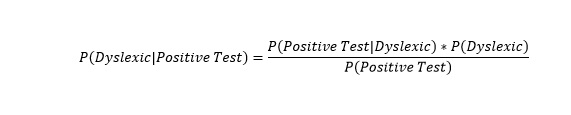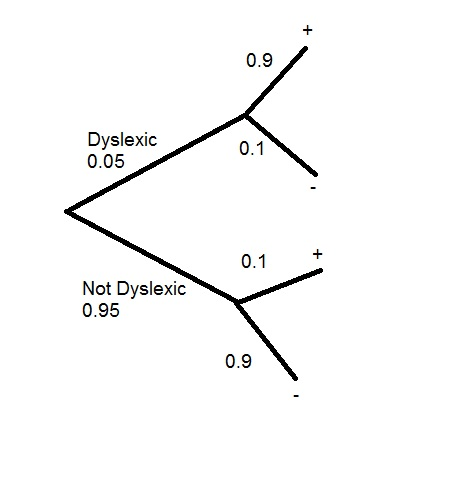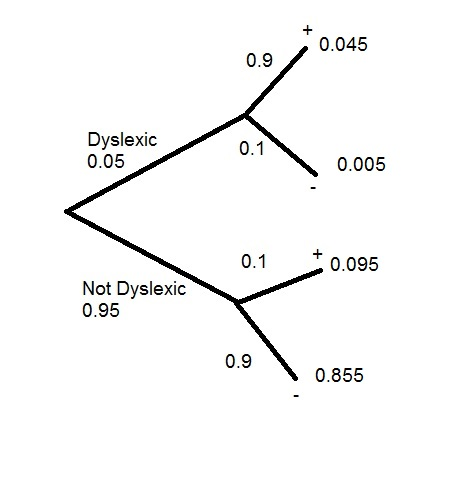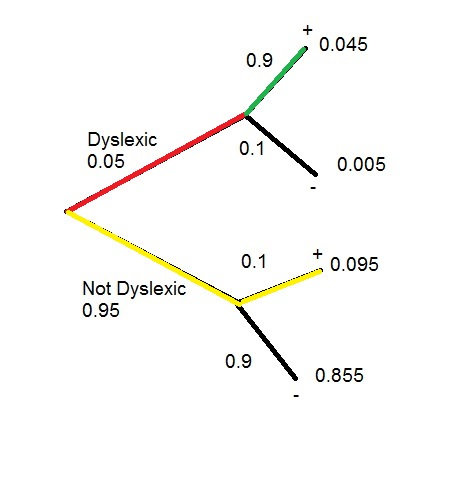# Bayes’ Theorem

Bayes’ Theorem sits at the heart of a few well known machine learning algorithms. So a fundamental understanding of the theorem is in order.

Let’s consider the following idea (the following stats are completely made up by the way). Imagine 5% of kids are dyslexic. Now imagine the tests administered for dyslexia at a local school is known to give a false positive 10% of the time. What is the probability a kid has dyslexia given the fact they tested positive?

What we want to know is = P(Dyslexic | Positive Test).

To figure this out, we are going to use Bayes’ TheoremDon’t worry. It is not all that complicated. Let’s break it down into parts:

• P(A) and P(B) are the probabilities of A or B happening independent of each other
• P(A|B) is the probability of A given the B has occurred
• P(B|A) is the probability of B given that A has occurred

Let’s take a new look at the formulaSo let me put this into English.

• P(Dyslexic|Positive Test) = probability the kid is dyslexic assuming he has positive test
• P(Dyslexic) = the probability the kid being dyslexic
• P(Positive Test) = Probability of a positive test
• P(Positive Test |Dyslexic) = The probability positive test assuming the kid is dyslexic

First, let’s figure out our probabilities. A tree chart is a great way to start.

Look at the chart below. It branches first between dyslexic and not dyslexic. Then each branch has positive and negative probabilities branching from there.Now to calculate the probabilities. We do this by multiplying the branches. For example Dyslexic and Positive  0.05 * 0.9 = 0.045Now, let’s fill in our formula. If you are having trouble seeing where the values come from look at the chart below

• P(Pos test | Dyslexic) = red * green = 0.05*0.9=.0.045
• P(Dyslexic) = First section of top branch = 0.05
• P(Positive Test) = red*green + yellow * yellow = 0.05*0.9+0.95*0.1=0.045+0.095So the probability of being dyslexic assuming the kid had a positive test = 0.016 or 1.6%

Another – perhaps more real world use for Bayes’ Theorem is the SPAM filter. Check it out below. See if you can figure your way through it on your own.• P(SPAM|Words) – probability an email is SPAM based on words found in the email
• P(SPAM) – probability of an email being SPAM in general
• P(Words) – probability of words appearing in email
• P(Words|SPAM) – probability of words being in an email if we know it is SPAM

## 4 thoughts on “Bayes’ Theorem”

1.agalea91

Nice example, I like how clearly you laid everything out. That being said I think the final probability (given a +ive test) of 1.6% is far too low. This is because the P(+ive|Dyslexic) = 0.9 and not 0.045 as you said.

1.Ben Larson

Great catch!!!! Curse of being a one man show, I don’t have anyone to double check my work. I will fix it over the weekend.

1.agalea91

I can’t believe how much content you put out Ben, it’s insane.

2.jnfdsnuc@gmail.com

wonderful points altogether, you just gained a brand new reader. What would you suggest in regards to your post that you made some days ago? Any positive?
Parajumpers Long Bear http://www.parajumpersjacketsale.com